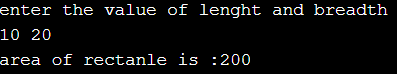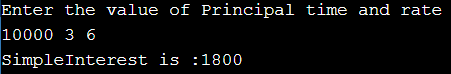# Assignment Solution of Operator and Expression

## Assignment Solution for Operator and Expression

In this article, I am going to give you the solutions that we give you as an assignment in our Operator and Expression article. So, please read our Operator and Expressions article if you have not read yet. First, try to solve the problem by yourself and then only look at the below solutions.

##### Question1: Program to find the area of a rectangle.

Solution: Before writing code first always we need to find the logic by writing an algorithm or pseudocode. Here the question is to find the area of a rectangle and from mathematics, we know the area of a rectangle is length*breadth i.e. area=l*b; now we have an expression to calculate the area.

##### Program:
```#include <iostream>
using namespace std;
int main ()
{
cout << "enter the value of lenght and breadth\n";
area = length * breadth;	//logic to calculate the area.
cout << "area of rectanle is :" << area << endl;
return 0;
}
```

Output:##### Question2: Program to calculate the Simple interest.

Solution: Same approach.
First logic: Simple interest=principal amount*time*rate of interest/100;
Simplified version: SI=P*T*R/100;(expression)

Program:

```#include <iostream>
using namespace std;
int main ()
{
int SimpleInterest, Principal, time, rate;
cout << "Enter the value of Principal time and rate\n";
cin >> Principal >> time >> rate;
SimpleInterest = (Principal * time * rate) / 100;
cout << "SimpleInterest is :" << SimpleInterest << endl;
return 0;
}
```

Output:That’s it. We have given the solutions. If you have a better solution, then please post your solution in the comment box so that other guys will get benefits.